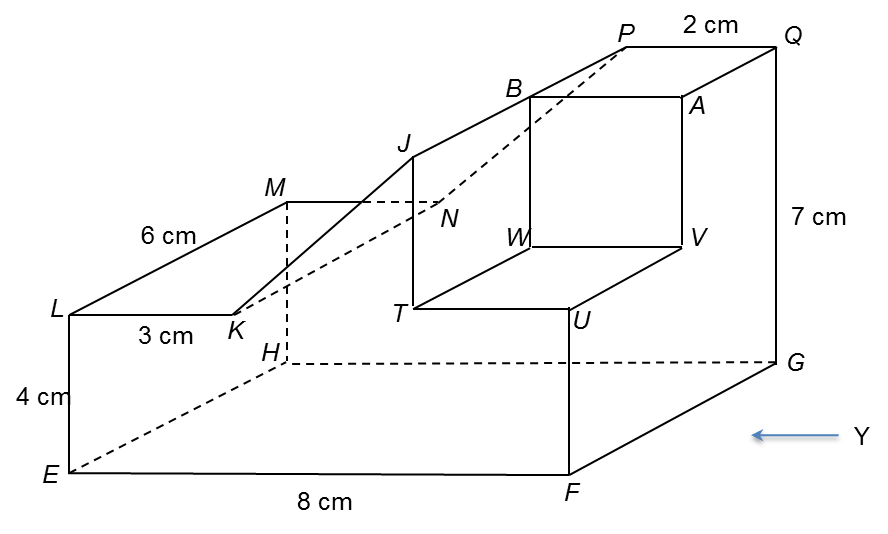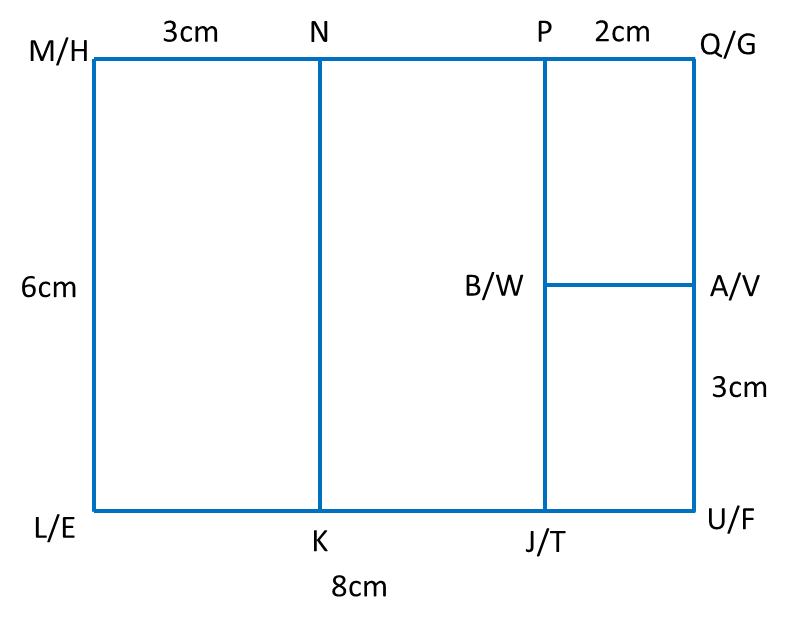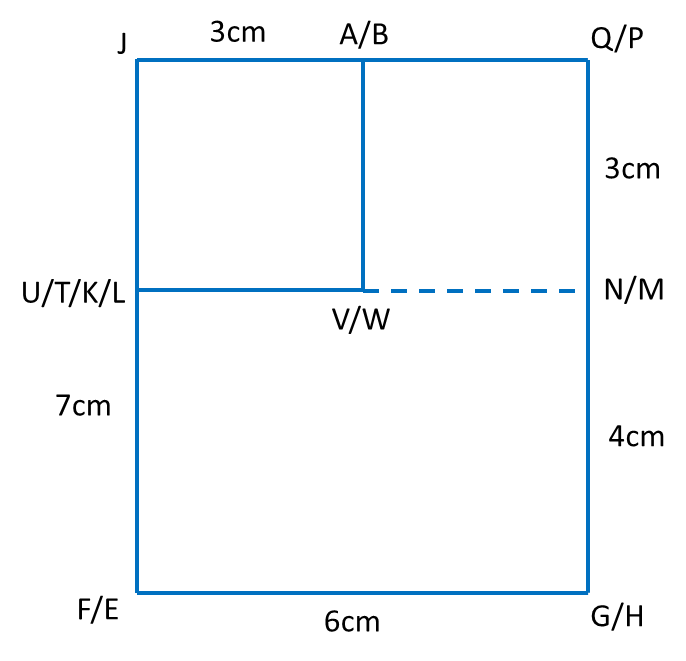# 7.3.7 Plans and Elevations, PT3 Focus Practice

 
  
  
   
Question 8:
 
You are not allowed to use graph paper to answer this question.  
 
  
   (a)  Diagram  8.1  shows a solid right prism with rectangular base EFGH  on a horizontal table.  The surface EFRJKL  is the uniform cross-section of the prism. Rectangle KLMN  and JPQR are horizontal planes.  Rectangle  JKNP  is an inclined plane. RF and LE  are vertical edges.Diagram 8.1
 
  

  
Draw full scale, the elevation of the solid on a vertical plane parallel to EF as viewed from  X                         [3 marks]
  
 
  
(b)  A solid cuboid is removed from the solid in Diagram 8.1. The remaining solid is as shown in Diagram 8.2. Rectangle TUVW is a horizontal plane. JT and BW are vertical edges. UF = 4 cm and UV= 3 cm.Diagram 8.2
  
  
Draw to full scale,
  
(i) the plan of the remaining solid. [4 marks]
   
(ii) the elevation of the remaining solid on a vertical plane parallel to FG  as viewed from Y. [5 marks]
   
 
  
Solution:
  
(a)(b)(i)(b)(ii)